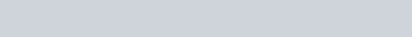In this video I give a worked example of the general solution for the second order linear differential equation of the form

General solutions of the form:

•In this example I show you how to find the general solution to

1.2nd Order Linear Differential Equations : P.I. = Exponential type : ExamSolutions - youtube Video